# Cylinder facts for kids

Kids Encyclopedia Facts

A cylinder is one of the most basic curved three dimensional geometric shapes, with the surface formed by the points at a fixed distance from a given line segment, known as the axis of the cylinder. The shape can be thought of as a circular prism. Both the surface and the solid shape created inside can be called a cylinder. The surface area and the volume of a cylinder have been known since ancient times.

In differential geometry, a cylinder is defined more broadly as a ruled surface which is spanned by a one-parameter family of parallel lines. A cylinder whose cross section is an ellipse, parabola, or hyperbola is called an elliptic cylinder, parabolic cylinder, or hyperbolic cylinder respectively.

## Common use

In common use a cylinder is taken to mean a finite section of a right circular cylinder, i.e., the cylinder with the generating lines perpendicular to the bases, with its ends closed to form two circular surfaces, as in the figure (right). If the cylinder has a radius r and length (height) h, then its volume is given by:

V = πr2h

and its surface area is:

• the area of the top r2) +
• the area of the bottom r2) +
• the area of the side (rh).

Therefore, without the top or bottom (lateral area), the surface area is:

A = 2πrh.

With the top and bottom, the surface area is:

A = 2πr2 + 2πrh = 2πr(r + h).

For a given volume, the cylinder with the smallest surface area has h = 2r. For a given surface area, the cylinder with the largest volume has h = 2r, i.e. the cylinder fits in a cube (height = diameter).

## Volume

Having a right circular cylinder with a height h units and a base of radius r units with the coordinate axes chosen so that the origin is at the center of one base and the height is measured along the positive x-axis. A plane section at a distance of x units from the origin has an area of A(x) square units where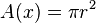$A(x)=\pi r^2$

or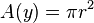$A(y)=\pi r^2$

An element of volume, is a right cylinder of base area Awi square units and a thickness of Δix units. Thus if V cubic units is the volume of the right circular cylinder, by Riemann sums,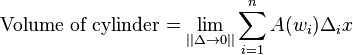$\mathrm{Volume \; of \; cylinder}=\lim_{||\Delta \to 0 ||} \sum_{i=1}^n A(w_i) \Delta_i x$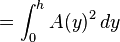$=\int_{0}^{h} A(y)^2 \, dy$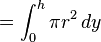$=\int_{0}^{h} \pi r^2 \, dy$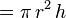$=\pi\,r^2\,h\,$

Using cylindrical coordinates, the volume can be calculated by integration over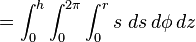$=\int_{0}^{h} \int_{0}^{2\pi} \int_{0}^{r} s \,\, ds \, d\phi \, dz$$=\pi\,r^2\,h\,$

## Cylindric section

Cylindric sections are the intersections of cylinders with planes. For a right circular cylinder, there are four possibilities. A plane tangent to the cylinder, meets the cylinder in a single straight line. Moved while parallel to itself, the plane either does not intersect the cylinder or intersects it in two parallel lines. All other planes intersect the cylinder in an ellipse or, when they are perpendicular to the axis of the cylinder, in a circle.

## Other types of cylinders

An elliptic cylinder, or cylindroid, is a quadric surface, with the following equation in Cartesian coordinates: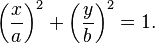$\left(\frac{x}{a}\right)^2+ \left(\frac{y}{b}\right)^2 = 1.$

This equation is for an elliptic cylinder, a generalization of the ordinary, circular cylinder (a = b). Even more general is the generalized cylinder: the cross-section can be any curve.

The cylinder is a degenerate quadric because at least one of the coordinates (in this case z) does not appear in the equation.

An oblique cylinder has the top and bottom surfaces displaced from one another.

There are other more unusual types of cylinders. These are the imaginary elliptic cylinders: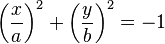$\left(\frac{x}{a}\right)^2 + \left(\frac{y}{b}\right)^2 = -1$

the hyperbolic cylinder: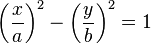$\left(\frac{x}{a}\right)^2 - \left(\frac{y}{b}\right)^2 = 1$

and the parabolic cylinder: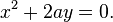$x^2 + 2ay = 0. \,$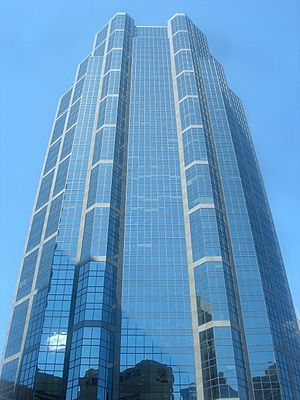In projective geometry, a cylinder is simply a cone whose apex is at infinity, which corresponds visually to a cylinder in perspective appearing to be a cone towards the sky.

## Projective geometry

In projective geometry, a cylinder is simply a cone whose apex is at infinity.

This is useful in the definition of degenerate conics, which require considering the cylindrical conics.

## Images for kidsCylinder Facts for Kids. Kiddle Encyclopedia.